# Boltzmann Statistics

## Boltzmann statistics

To describe a system consisting of a large number of particles in a physically useful manner, recourse must be had to so-called statistical procedures. If the mechanical laws operating in the system are those of classical mechanics, and if the system is sufficiently dilute, the resulting statistical treatment is referred to as Boltzmann or classical statistics. (Dilute in this instance means that the total volume available is much larger than the proper volume of the particles.) A gas is a typical example: The molecules interacting according to the laws of classical mechanics are the constituents of the system, and the pressure, temperature, and other parameters are the overall entites which determine the macroscopic behavior of the gas. In a case of this kind it is neither possible nor desirable to solve the complicated equations of motion of the molecules; one is not interested in the position and velocity of every molecule at any time. The purpose of the statistical description is to extract from the mechanical description just those features relevant for the determination of the macroscopic properties and to omit others.

The basic notion in the statistical description is that of a distribution function. Suppose a system of N molecules is contained in a volume V. The molecules are moving around, colliding with the walls and with each other. Construct the following geometrical representation of the mechanical system. Introduce a six-dimensional space (usually called the μ space), three of its coordinate axes being the spatial coordinates of the vessel x, y, z, and the other three indicating cartesian velocity components vx, vy, vz. A molecule at a given time, having a specified position and velocity, may be represented by a point in this six-dimensional space. The state of the gas, a system of N molecules, may be represented by a cloud of N points in this space. In the course of time, this cloud of N points moves through the μ space.

Note that the μ space is actually finite; the coordinates x, y, z of the molecules' position are bounded by the finite size of the container, and the velocities are bounded by the total energy of the system. Imagine now that the space is divided into a large number of small cells, of sizes w1,…, wi,…. A certain specification of the state of the gas is obtained if, at a given time t, the numbers n1(t),…, ni(t),… of molecules in the cells 1,…, i, … are given. To apply statistical methods, one must choose the cells such that on the one hand a cell size w is small compared to the macroscopic dimensions of the system, while on the other hand w must be large enough to allow a large number of molecules in one cell. If the cells are thus chosen, the numbers ni(t), the occupation numbers, will be slowly changing functions of time. The distribution functions fi(t) are defined by Eq. (1). (1)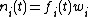The distribution function fi describes the state of the gas, and fi of course varies from cell to cell. Since a cell i is characterized by a given velocity range and position range, and since for appropriately chosen cells f should vary smoothly from cell to cell, f is often considered as a continuous function of the variables x, y, z, vx, vy, vz. The cell size w then can be written as dxdydzdvxdvydvz.

Since a cell i determines both a position and a velocity range, one may associate an energy εi with a cell. This is the energy a single molecule possesses when it has a representative point in cell i. This assumes that, apart from instantaneous collisions, molecules exert no forces on each other. If this were not the case, the energy of a molecule would be determined by the positions of all other molecules.

Most of the physically interesting quantities follow from a knowledge of the distribution function; the main problem in Boltzmann statistics is to find out what this function is. It is clear that ni(t) changes in the course of time for three reasons: (1) Molecules located at the position of cell i change their positions and hence move out of cell i; (2) molecules under the influence of outside forces change their velocities and again leave the cell i; and (3) collisions between the molecules will generally cause a (discontinuous) change of the occupation numbers of the cells. Whereas the effect of (1) and (2) on the distribution function follows directly from the mechanics of the system, a separate assumption is needed to obtain the effect of collisions on the distribution function. This assumption, the collision-number assumption, asserts that the number of collisions per unit time, of type (i, j) → (k, l) [molecules from cells i and j collide to produce molecules of different velocities which belong to cells k and l], called Aijkl, is given by Eq. (2).

(2)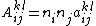Here aijkl depends on the collision configuration and on the size and kind of the molecules but not on the occupation numbers. Gains and losses of the molecules in, say, cell i can now be observed. If the three factors causing gains and losses are combined, the Boltzmann transport equation, written as Eq. (3),
(3)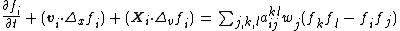is obtained. Here Δxfi is the gradient of f with respect to the positions, refers similarly to the velocities, and X i is the outside force per unit mass at cell i. This nonlinear equation determines the temporal evolution of the distribution function. Exact solutions are difficult to obtain. Yet Eq. (3) forms the basis for the kinetic discussion of most transport processes. There is one remarkable general consequence, which follows from Eq. (3). If one defines H(t) as in Eq. (4), one finds by straight manipulation from Eqs. (3) and (4)
(4)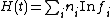(5)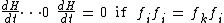that Eqs. (5) hold. Hence H is a function which in the course of time always decreases. This result is known as the H theorem. The special distribution which is characterized by Eq. (6)
(6)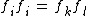has the property that collisions do not change the distribution in the course of time; it is an equilibrium or stationary distribution.

The form of the equilibrium distribution may be determined from Eq. (6), with the help of conservation laws. For a gas which as a whole is at rest, it may be shown that the only solution to functional Eq. (6) is given by Eqs. (7a) or (7b). Here A

(7a)(7b)and ß are parameters, not determined by Eq. (6), and U is the potential energy at the point x, y, z. Equations (7a) and (7b) are the Maxwell-Boltzmann distribution. Actually A and ß can be determined from the fact that the number of particles and the energy of the system are specified.

The indiscriminate use of the collision-number assumption leads, via the H theorem, to paradoxical results. The basic conflict stems from the irreversible results that appear to emerge as a consequence of a large number of reversible fundamental processes. A careful treatment of the explicit and hidden probability assumptions is the key to the understanding of the apparent conflict. The equilibrium distribution may be thought of as the most probable state of a system. If a system is not in equilibrium, it will most likely (but not certainly) go there; if it is in equilibrium, it will most likely (but not certainly) stay there. By using such probability statements, it may be shown that the paradoxes and conflicts may indeed be removed. A consequence of the probabilistic character of statistics is that the entities computed also possess this characteristic. For example, one cannot really speak definitively of the number of molecules hitting a section of the wall per second, but only about the probability that a given number will hit the wall, or about the average number hitting. In the same vein, the amount of momentum transferred to a unit area of the wall by the molecules per second (this, in fact, is precisely the pressure) is also to be understood as an average. This in particular means that the pressure is a fluctuating entity. The fluctuations in pressure may be demonstrated by observing the motion of a mirror, suspended by a fiber, in a gas. On the average, as many gas molecules will hit the back as the front of the mirror, so that the average displacement will indeed be zero. However, it is easy to imagine a situation where more momentum is transferred in one direction than in another, resulting in a deflection of the mirror. From the knowledge of the distribution function the probabilities for such occurrences may indeed be computed; the calculated and observed behavior agree very well. This clearly demonstrates the essentially statistical character of the pressure. See Boltzmann transport equation, Brownian movement, Kinetic theory of matter, Quantum statistics, Statistical mechanics

McGraw-Hill Concise Encyclopedia of Physics. © 2002 by The McGraw-Hill Companies, Inc.
The following article is from The Great Soviet Encyclopedia (1979). It might be outdated or ideologically biased.

## Boltzmann Statistics

a physical statistics for systems of a large number of noninteracting particles. Strict Boltzmann statistics governs atomic and molecular ideal gases—that is, gases in which the potential energy of molecular interaction is considered as negligible. In reality, such systems include rarefied gases, whose molecules interact weakly with each other.

It is not possible to describe in detail the behavior of each particle for a system having a large number of particles. However, the general features of the system’s behavior as a whole are the averaged reflection of the motions of individual particles. The particles are distributed over the states possible for them; their position coordinates r and momenta p take on specific values. This is described mathematically by a distribution function, which characterizes the probability that a particle will be found in a given state.

For an ideal gas composed of molecules which exist in an external force field, the Boltzmann distribution function has the form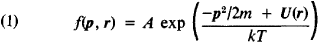where p2/2m is the kinetic energy of a molecule of mass m, V(r) is its potential energy in an external field, k is the Boltzmann constant, and T is the absolute temperature of the gas; the constant/! is determined from the condition that the total number of particles, distributed over all possible states, is equal to the total number of particles in the system (the normalization condition). Since the quantity kT characterizes the average energy of a molecule’s thermal motion, the distribution of particles among the states in Boltzmann statistics is determined by the ratio of the total energy of a particle (kinetic and potential) to the energy of its thermal motion.

Distribution function (1) contains two factors: exp(-p2/2mkT) and exp(-U(r)/kT). The first term determines the distribution of molecules by momentum (or velocity)—that is, it is the Maxwell distribution—and the second determines the distribution by position coordinates in an external force field. Therefore, the second function alone is sometimes called the Boltzmann distribution, and formula (1) is called the Maxwell-Boltzmann distribution.

Using the Boltzmann distribution, it is easy to obtain the formula for the dependence of the concentration of air molecules (independent of their momenta) on the altitude above the earth’s surface and, consequently, the barometric formula, which defines the dependence of air pressure on altitude.

In quantum statistics, instead of a distribution function, the average number of particles ň, found in a given quantum state with an energy is considered, and the Boltzmann distribution takes the form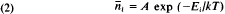The constant A is found from the condition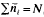, where N is the total number of particles in the system and is equal to A = (N/V) (h2lmkT)3/2. (V is the volume of the gas; h is Planck’s constant.) Distribution (2) is the limiting case of Bose-Einstein and Fermi-Dirac quantum statistics when it is possible to disregard the quantum-mechanical effects connected with the mutual influence of identical particles. It holds true for systems in which all the numbers ñ¡ are small compared with 1; this indicates that the particles spend almost the whole time in strongly differing states, and consequently, their specific influence on each other does not appear.

Quantum Boltzmann statistics holds true for low gas densities N/V and high temperatures (for a given mass of particles). In practice, Boltzmann statistics is applicable to all rarefied molecular gases, since the mass of the molecules is great and the quantum effect of identical particles on each other should only appear at the high densities and low temperatures that correspond to the solid state (for helium, the liquid state) of matter. (In this case Boltzmann statistics is entirely inapplicable, since the interaction of the molecules is great.) Boltzmann statistics is not applicable to an electronic gas in metals or a gas of light quanta (photons).

V. P. PAVLOV

The Great Soviet Encyclopedia, 3rd Edition (1970-1979). © 2010 The Gale Group, Inc. All rights reserved.

## Boltzmann statistics

[′bōlts·mən stə′tis·tiks]
(statistical mechanics)
McGraw-Hill Dictionary of Scientific & Technical Terms, 6E, Copyright © 2003 by The McGraw-Hill Companies, Inc.
References in periodicals archive ?
The data, consisting of the number of balls escaping the central well at different forcing energies, exhibit a logarithmic dependence on the reciprocal of the applied energy (analogous to thermal energy [k.sub.B]T) that is similar to that given by Boltzmann statistics and the Clausius-Clapeyron equation.
Physical analogies have been used, for instance, by Prentis (2000) to illustrate how mechanical systems with "motorized molecules" can be described well by Boltzmann statistics when using the ergodic hypothesis of statistical mechanics.
Such a kinetic viewpoint is consistent with chemical kinetics and Boltzmann statistics, as seen by taking the logarithm of Eq.
The data from balls jumping out of a potential well in this mechanical system can be interpreted further with the help of Boltzmann statistics. An underlying feature of classical statistical mechanics is the fact that all energy states are equally likely, so a closed system overall tends toward the most probable distribution.
The fact that this mechanical system obeys Boltzmann statistics so well may arise from the chaotic nature of particle-particle collisions and even of particle-plate interactions when many impacts occur per second.
The mechanical system has a behavior predicted by Boltzmann statistics and therefore also illustrates the essential physics of the Clausius-Clapeyron equation.
(4) and (12) that the second virial coefficient B(T) and the third virial coefficient C(T) for a quantum system obeying Boltzmann statistics can be written as
At low temperatures where Boltzmann statistics is no longer a good approximation, the above equations must be extended to incorporate Bose-Einstein or Fermi-Dirac statistics.
In the following, we will not be concerned with temperatures so low that exchange effects play a relevant role  and hence we will approximate the sum over [[^.P].sub.[pi]] with the identity operator (Boltzmann statistics).
(14) in the case of Boltzmann statistics, is readily obtained by first performing a canonical transformation to the center of mass R [equivalent to] [([x.sub.1] + [x.sub.2)]/2] and relative r [equivalent to] [r.sub.1] [equivalent to] [x.sub.2] - [x.sub.1] coordinates.

Site: Follow: Share:
Open / Close